Function Repository Resource:

# ComplementaryAngle

Compute the geometric complement to a given angle

Contributed by: Paco Jain (Wolfram Research)
 ResourceFunction["ComplementaryAngle"][θ] gives the complement to the angle of θ.

## Details and Options

ResourceFunction["ComplementaryAngle"] takes all angles to be measured in radians.
The complement of an angle θ is defined to be the angle which sums with θ to form a right angle. In other words,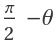.

## Examples

### Basic Examples (1)

Compute the complement of an angle:

 In:=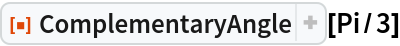Out=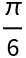### Scope (1)

ComplementaryAngle works directly on Quantity expressions with units of "Radians":

 In:=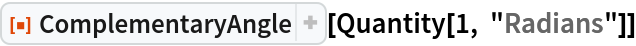Out=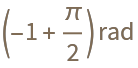### Properties and Relations (4)

ComplementaryAngle will remain unevaluated for non-numeric input:

 In:=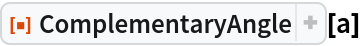Out=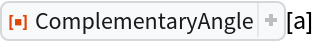ComplementaryAngle will return a negative result if the input angle is greater than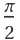:

 In:=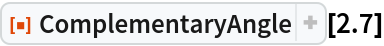Out=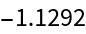For negative input, ComplementaryAngle returns a result greater than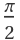:

 In:=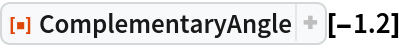Out=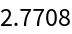For numeric input, ComplementaryAngle is its own inverse:

 In:=Out=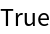## Version History

• 1.0.0 – 05 July 2019